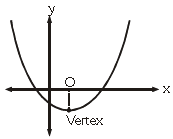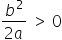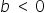Maths-
General
Easy

Question

# Graph of y = ax2 + bx + c = 0 is given adjacently. What conclusions can be drawn from this graph –## a > 0    b < 0    c < 0    All of the aboveHint:

## The correct answer is: All of the above

### As we can see from the graph we have a parabola curve and since it is opening in an upward direction. So we can say that a > 0 andHence, the option (a) is correct.Here, we can see that the vertex of the parabola is located in the fourth quadrant , therefore it will be =On further solving this, we getTherefore, the option (b) is also correct.Since, at x=0 , the y intercept will be positive and from this, we can conclude that c < 0 andHence, the option (c) will also be correctOn checking all the options, and we can see all options are correct andTherefore, we conclude that all the options available are correct.

Here we can see that the graph was given to us and us to take out the conclusion from that since we have the options available so I would suggest you to always start to check from the options because by the use of options we can see how easily we concluded this question.

### Related Questions to study#### With Turito Foundation.#### Get an Expert Advice From Turito.